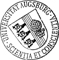Asymptotic Goodness-of-Fit Tests for Point Processes Based on Scaled Empirical K-Functions

• We study sequences of scaled edge-corrected empirical (generalized) K-functions (modifying Ripley's K-function) each of them constructed from a single observation of a d-dimensional fourth-order stationary point process in a sampling window W_n which grows together with some scaling rate unboundedly as n --> infty. Under some natural assumptions it is shown that the normalized difference between scaled empirical and scaled theoretical K-function converges weakly to a mean zero Gaussian process with simple covariance function. This result suggests discrepancy measures between empirical and theoretical K-function with known limit distribution which allow to perform goodness-of-fit tests for checking a hypothesized point process based only on its intensity and (generalized) K-function. Similar test statistics are derived for testing the hypothesis that two independent point processes in W_n have the same distribution without explicit knowledge of their intensities and K-functions.

Author: Lothar HeinrichGND urn:nbn:de:bvb:384-opus4-41906 https://opus.bibliothek.uni-augsburg.de/opus4/4190 Preprints des Instituts für Mathematik der Universität Augsburg (2017-01) Preprint English Universität Augsburg 2017/04/07 fourth-order Brillinger-mixing; generalized K-function; edge-corrected empirical K-function; scaling rate; Skorohod-space; functional central limit theorem; one- and two-sample tests for point processes Anpassungstest; Punktprozess; Zentraler Grenzwertsatz Mathematisch-Naturwissenschaftlich-Technische Fakultät Mathematisch-Naturwissenschaftlich-Technische Fakultät / Institut für Mathematik Mathematisch-Naturwissenschaftlich-Technische Fakultät / Institut für Mathematik / Lehrstuhl für Stochastik und ihre Anwendungen 5 Naturwissenschaften und Mathematik / 51 Mathematik / 510 MathematikDeutsches Urheberrecht mit Print on Demand